+
Yield
Time Value of Money and Financial Securities
0
of 0 possible points

# Yield

Author: Sophia Tutorial
##### Description:

Differentiate between the methods of calculating yield of a single period investment and an annuity.

(more)### Developing Effective Teams

*No strings attached. This college course is 100% free and is worth 1 semester credit.

37 Sophia partners guarantee credit transfer.

299 Institutions have accepted or given pre-approval for credit transfer.

* The American Council on Education's College Credit Recommendation Service (ACE Credit®) has evaluated and recommended college credit for 32 of Sophia’s online courses. Many different colleges and universities consider ACE CREDIT recommendations in determining the applicability to their course and degree programs.

Tutorial

what's covered
In this lesson, you will differentiate between the methods of calculating yield of a single-period investment and an annuity. Specifically, this lesson will cover:
1. Calculating the Yield of a Single-Period Investment
1. Change-In-Value
2. Annual Percentage Rate
3. Effective Annual Rate
2. Calculating the Yield of an Annuity

## 1. Calculating the Yield of a Single-Period Investment

The yield on an investment is the amount of money that is returned to the owner at the end of the term. In short, it’s how much you get back on your investment.

Naturally, this is a number that people care a lot about. The whole point of making an investment is to get a yield. There are a number of different ways to calculate an investment’s yield, though. You may get slightly different numbers using different methods, so it’s important to make sure that you use the same method when you are comparing yields. This section will address the yield calculation methods you are most likely to encounter, though there are many more.

term to know

Yield
The amount in cash that returns to the owners of a security.
1a. Change-In-Value
The most basic type of yield calculation is the change-in-value calculation. This is simply the change in value (FV minus PV) divided by the PV times 100%. This calculation measures how different the FV is from the PV as a percentage of PV.

formula

Change-in-Value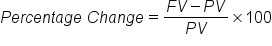1b. Annual Percentage Rate
Another common way of calculating yield is to determine the Annual Percentage Rate, or APR. You may have heard of APR from ads for car loans or credit cards. These generally have monthly loans or fees, but if you want to get an idea of how much you will accrue in interest per year, you need to calculate an APR. Nominal APR is simply the interest rate multiplied by the number of payment periods per year.

formula

Nominal Annual Percentage Rate (APR)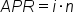However, since interest compounds, nominal APR is not a very accurate measure of the amount of interest you actually accrue.

1c. Effective Annual Rate
The Effective Annual Rate is the amount of interest actually accrued per year based on the APR. The following formula can be used, where n is the number of compounding periods of APR per year:

formula
Effective Annual Rate (EAR)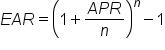### IN CONTEXT

You may see an ad that says you can get a car loan at an APR of 10% compounded monthly. That means that the APR is 0.10 and n is 12, since the APR compounds 12 times per year. The effective APR would then be: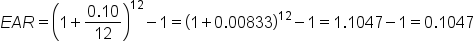That means the EAR is 10.47%.

The EAR is a form of the Annual Percentage Yield (APY). APY may also be calculated using interest rates other than APR, so the following is a more general formula:

formula

Annual Percentage Yield (APY)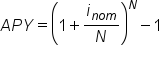The logic behind calculating APY is the same as that used when calculating EAR: you want to know how much you actually accrue in interest per year. Interest usually compounds, so there is a difference between the nominal interest rate (e.g., monthly interest times 12) and the effective interest rate.

big idea
The Annual Percentage Yield is a way of normalizing the nominal interest rate. Basically, it is a way to account for the time factor in order to get a more accurate number for the actual interest rate In the formula, inom is the nominal interest rate and N is the number of compounding periods per year.

## 2. Calculating the Yield of an Annuity

The yield of an annuity can be calculated in similar ways to the yield for a single payment, but two methods are most common.

• Percentage-Change: Just as for a single payment, this method calculates the percentage difference between the FV and the PV. Since annuities include multiple payments over the lifetime of the investment, the PV is the present value of the entire investment, not just the first payment.
• Internal rate of return (IRR): This method is the interest rate (or discount rate) that causes the Net Present Value (NPV) of the annuity to equal 0. That means that the PV of the cash outflows equals the PV of the cash inflows. The higher the IRR, the more desirable is the investment. In theory, you should make investments with an IRR greater than the cost of capital.

### IN CONTEXT

Suppose you have a potential investment that would require you to make a \$4,000 investment today, but would return cash flows of \$1,200, \$1,410, \$1,875, and \$1,050 in the four successive years. This investment has an implicit rate of return, but you don’t know what it is. You plug the numbers into the NPV formula and set NPV equal to 0.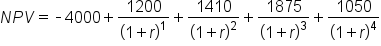You then solve for r, which is your IRR. It is not easy to solve this problem by hand. You will likely need to use a business calculator or Excel. When r = 14.3%, NPV = 0, so therefore the IRR of the investment is 14.3%.

hint
This above example is not technically an annuity because the payments vary, but it still is a good example for how to find IRR.

summary
In this lesson, you learned that there are different methods of calculating the yield of a single-period investment, such as determining the change-in-value, the annual percentage rate, or the effective annual rate. Similarly, calculating the yield of an annuity can be done in different ways, most commonly by determining the percentage-change or the internal rate of return.

Best of luck in your learning!

Source: THIS CONTENT HAS BEEN ADAPTED FROM LUMEN LEARNING'S “Yield” TUTORIAL.

Terms to Know
Yield

The amount in cash that returns to the owners of a security.

Formulas to Know
Annual Percentage Yield (APY)Change-in-ValueEffective Annual Rate (EAR)Nominal Annual Percentage Rate (APR)Rating